Share

# Frank solutions for Class 10 Maths chapter 16 - Loci

#### Frank Frank Class 10 Mathematics Part 2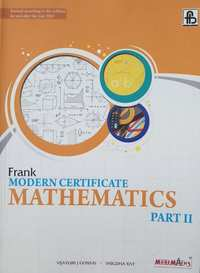## Chapter 16: Loci

Exercise 16.1Exercise 15.1

#### Frank solutions for Class 10 Maths Chapter 16 Exercise Exercise 16.1, Exercise 15.1 [Page 0]

Draw a straight line AB of 9 cm. Draw the locus of all points which are equidistant from A and B. Prove your statement.

Two straight roads AB and CD cross each other at Pat an angle of 75°  . X is a stone on the road AB, 800m from P towards B. BY taking an appropriate scale draw a figure to locate the position of a pole, which is equidistant from P and X, and is also equidistant from the roads.

Draw two intersecting lines to include an angle of 30°  . Use ruler and compasses to I ocate points which a re e qui distant from these Ii ne s and also 2 cm away from their point of intersection. How many such points exist ?

AB and CD are two intersecting lines. Find a point equidistant from AB and CD, and also at a distance of 1.8 cm from another given line EF.

Without using set squares or protractor, construct a quadrilateral ABCD in which ∠ BAD = 45° , AD = AB = 6 cm, BC= 3.6 cm and CD=5 cm. Locate the point P on BD which is equidistant from BC and CD.

Construct a rhombus ABCD with sides of length 5 cm and diagonal AC of length 6 cm. Measure ∠ ABC. Find the point R on AD such that RB = RC. Measure the length of AR.

Construct a rhombus ABCD whose diagonals AC and BD are 8 cm and 6 cm respectively. Find by construction a point P equidistant from AB and AD and also from C and D.

Construct a ti.PQR, in which PQ=S. 5 cm, QR=3. 2 cm and PR=4.8 cm. Draw the locus of a point which moves so that it is always 2.5 cm from Q.

Construct a Δ XYZ in which XY= 4 cm, YZ = 5 cm and ∠ Y = 1200. Locate a point T such that ∠ YXT is a right angle and Tis equidistant from Y and Z. Measure TZ.

In  Δ PQR, s is a point on PR such that ∠ PQS = ∠  RQS . Prove thats is equidistant from PQ and QR.

In the given figure ABC is a triangle. CP bisects angle ACB and MN is perpendicular bisector of BC. MN cuts CP at Q. Prove Q is equidistant from B and C, and also that Q is equidistant from BC and AC.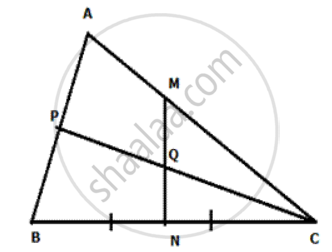A and B are fixed points while Pis a moving point, moving in a way that it is always equidistant from A and B. What is the locus of the path traced out by the pcint P?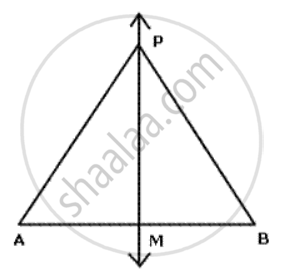In given figure, ABCD is a kite. AB = AD and BC =CD. Prove that the diagona AC is the perpendirular bisector of the diagonal BD.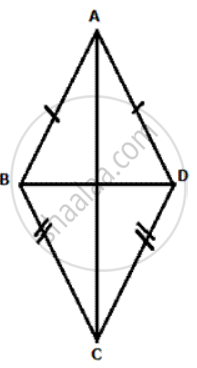In given figure 1 ABCD is an arrowhead. AB = AD and BC = CD. Prove th at AC produced bisects BD at right angles at the point M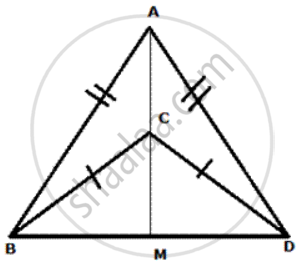In Δ PQR, bisectors of  ∠ PQR and ∠ PRQ meet at I. Prove that I is equidistant from the three sides of the triangle , and PI bisects ∠ QPR .

In  Δ ABC, the perpendicular bisector of AB and AC meet at 0. Prove that O is equidistant from the three vertices. Also, prove that if M is the mid-point of BC then OM meets BC at right angles.

In a quadrilateral PQRS, if the bisectors of ∠ SPQ and ∠ PQR meet at O, prove that O is equidistant from PS and QR.

In a quadrilateral ABCD, if the perpendicular bisectors of AB and AD meet at P, then prove that BP = DP.

In Δ ABC, B and Care fixed points. Find the locus of point A which moves such that the area of Δ ABC remains the same.

A point moves such that its distance from a fixed line AB is always the same. What is the relation between AB and the path travelled by the point?

State the locus of a point moving so that its perpendicular distances from two given lines is always equal.

AB is a fixed line) state the locus of the point P such that ∠ APB = 90° .

Draw and describe the lorus in  the following cases:

The locus of points at a distance of 4 cm from a fixed line.

Draw and describe the lorus in the following cases:

The lorus of points inside a circle and equidistant from two fixed points on the circle .

Draw and describe the lorus in the following cases:

The Iocus of the mid-points of all parallel chords of a circle.

Draw and describe the lorus in the following cases:

The lorus of a point in rhombus ABCD which is equidistant from AB and AD .

Draw and describe the locus in the following cases :

The locus of a point in the rhombus ABCD which is equidistant from the point  A and C

Describe completely the locus of point in  the following cases:

Midpoint of radii of a circle.

Describe completely the locus of point in the following cases:

Centre of a ball, rolling along a straight line on a level floor.

Describe completely the locus of points in the following cases:

Point in a plane equidistant from a given line.

Describe completely the locus of points in the following cases:

Centre of a circle of varying radius and touching the two arms of ∠ ABC.

Describe completely the locus of points in the following cases:

Centre of a cirde of radius 2 cm and touching a fixed circle of radius 3 cm with centre O.

Using only ruler and compasses, construct a triangle ABC 1 with AB = 5 cm, BC = 3.5 cm and AC= 4 cm. Mark a point P, which is equidistant from AB, BC and also from Band C. Measure the length of PB.

Construct a triangle ABC, such that AB= 6 cm, BC= 7.3 cm and CA= 5.2 cm. Locate a point which is equidistant from A, B and C.

## Chapter 16: Loci

Exercise 16.1Exercise 15.1

#### Frank Frank Class 10 Mathematics Part 2## Frank solutions for Class 10 Mathematics chapter 16 - Loci

Frank solutions for Class 10 Maths chapter 16 (Loci) include all questions with solution and detail explanation. This will clear students doubts about any question and improve application skills while preparing for board exams. The detailed, step-by-step solutions will help you understand the concepts better and clear your confusions, if any. Shaalaa.com has the CISCE Frank Class 10 Mathematics Part 2 solutions in a manner that help students grasp basic concepts better and faster.

Further, we at Shaalaa.com provide such solutions so that students can prepare for written exams. Frank textbook solutions can be a core help for self-study and acts as a perfect self-help guidance for students.

Concepts covered in Class 10 Mathematics chapter 16 Loci are Introduction of Loci, Loci Examples, Constructions Under Loci, Theorems Based on Loci.

Using Frank Class 10 solutions Loci exercise by students are an easy way to prepare for the exams, as they involve solutions arranged chapter-wise also page wise. The questions involved in Frank Solutions are important questions that can be asked in the final exam. Maximum students of CISCE Class 10 prefer Frank Textbook Solutions to score more in exam.

Get the free view of chapter 16 Loci Class 10 extra questions for Maths and can use Shaalaa.com to keep it handy for your exam preparation

S